# Rounding Numbers to Millions in Excel

To round the numbers to Millions we can use Custom Format and Round function in Excel.

We can round to the nearest million calculation from the 2 different ways.

1st Custom Formatting

2nd Round Function
Custom Format: -We can use Custom Number Format, to display a Number in Million or Display it in some Color. It’s a tool from in old versions of excel when there was no Conditional Formatting option in Excel.All conditional options are performed by using Custom Number Formal.
ROUND: - This function is used to round a number to a specified number of digits.

Syntax of “ROUND” function:       =ROUND (number, num_digits)

Example:Cell A1 contains the number 456.25

=ROUND(A1, 0), function will return456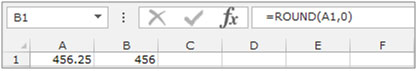Round the number by using the Custom Format

Let’s take an example and understand how we can round the numbers to Millions.

We have categorized Income data. In which column D contains City Name, column E contains Gender and column F contains Income in \$. We want to format income in Millions.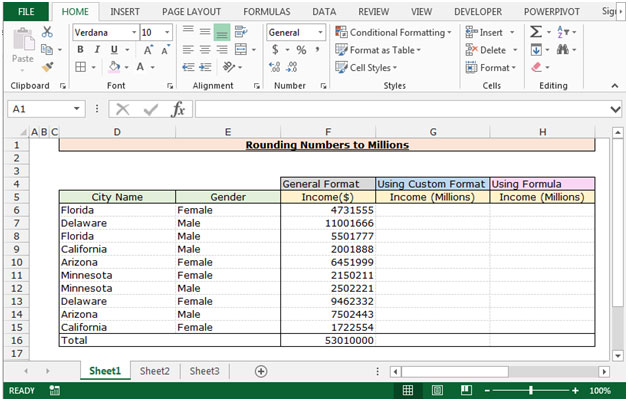To Round the income in Millions follow below given steps:-

• Select the range F6:F16 and copy by pressing the key Ctrl+C and paste in the range G6:G16 by pressing the key Ctrl+V on your keyboard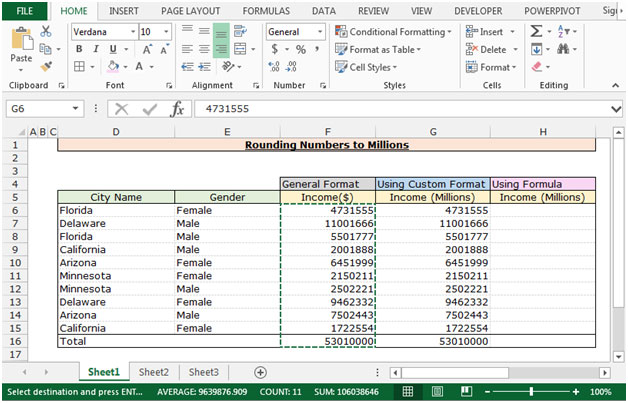• Select the range G6:G16 and press the key Ctrl+1 on your keyboard.
• Format Cells dialog box will appear.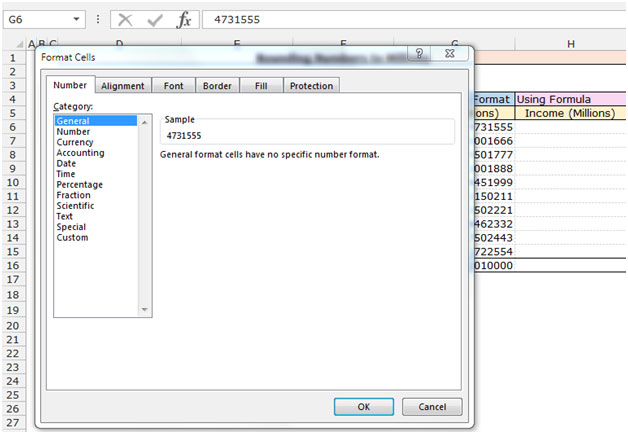• In the Number Tab, Click on Custom.
• After clicking on the Custom, related options will get appear.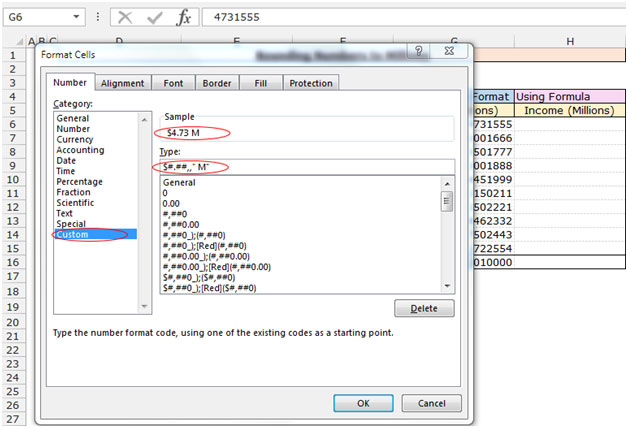• In the type box write the format to round the number in thousands \$#.##,,"M";
• The function will return the rounded figures in Millions.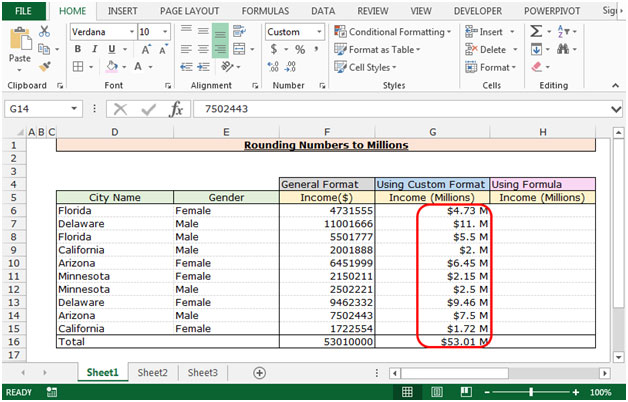Round the number by using the Round Function

Follow below given steps:-

• Select the Cell H6 and write the formula.
• ="\$"&ROUND((F6/1000000),2)&"M"press enter.
• The function will round the number in Millions.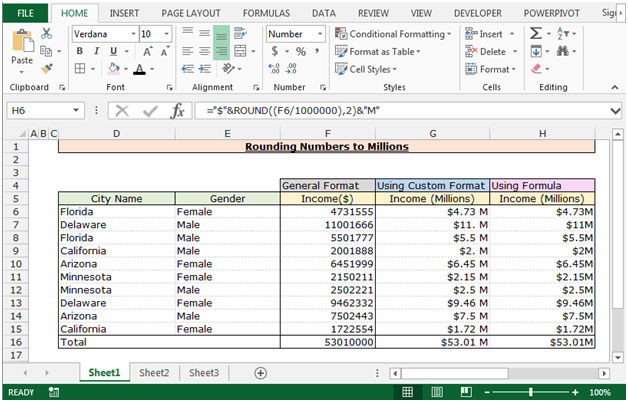This is the way we can round the number in Millions by using the Round formula and Custom Format in Microsoft Excel.

1.